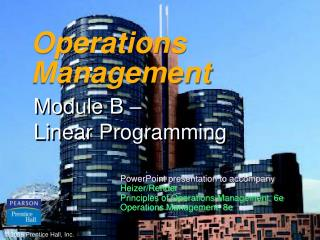DownloadDownload PresentationOperations Management

# Operations Management

Download Presentation## Operations Management

- - - - - - - - - - - - - - - - - - - - - - - - - - - E N D - - - - - - - - - - - - - - - - - - - - - - - - - - -
##### Presentation Transcript

1. Operations Management Module B – Linear Programming PowerPoint presentation to accompany Heizer/Render Principles of Operations Management, 6e Operations Management, 8e © 2006 Prentice Hall, Inc.

2. Lecture Outline • More Examples • Practice formulating • Practice solving • Practice interpreting the results

3. Mixed Nuts • Crazy Joe makes two blends of mixed nuts: party mix and regular mix. • Crazy Joe has 10 lbs of cashews and 24 lbs of peanuts • Crazy Joe wants to maximize revenue. Please help him.

4. Ah, Nuts Formulation • Let • p = lbs of party mix to make • r = lbs of regular mix to make • z = total revenue • Max z = 6p + 4r • Subject to • 0.6p + 0.9r< 24 (peanut constraint) • 0.4p + 0.1r< 10 (cashew constraint) • p, r> 0 (non-negativity constraints)

5. Solving Minimization Problems • Formulated and solved in much the same way as maximization problems • In the graphical approach an iso-cost line is used • The objective is to move the iso-cost line inwards until it reaches the lowest cost corner point

6. Minimization Example Let X1 = number of tons of black-and-white chemical produced X2 = number of tons of color picture chemical produced Minimize total cost = 2,500X1 + 3,000X2 Subject to: X1 ≥ 30 tons of black-and-white chemical X2 ≥ 20 tons of color chemical X1 + X2≥ 60 tons total X1, X2≥ 0 nonnegativity requirements

7. X2 60 – 50 – 40 – 30 – 20 – 10 – – X1 + X2= 60 X1= 30 X2= 20 | | | | | | | 0 10 20 30 40 50 60 X1 Minimization Example Table B.9 Feasible region b a

8. Minimization Example Total cost at a = 2,500X1 + 3,000X2 = 2,500 (40) + 3,000(20) = \$160,000 Total cost at b = 2,500X1 + 3,000X2 = 2,500 (30) + 3,000(30) = \$165,000 Lowest total cost is at point a

9. Feed Product Stock X Stock Y Stock Z A 3 oz 2 oz 4 oz B 2 oz 3 oz 1 oz C 1 oz 0 oz 2 oz D 6 oz 8 oz 4 oz LP Applications Diet Problem Example

10. LP Applications X1 = number of pounds of stock X purchased per cow each month X2 = number of pounds of stock Y purchased per cow each month X3 = number of pounds of stock Z purchased per cow each month Minimize cost = .02X1 + .04X2 + .025X3 Ingredient A requirement:3X1 + 2X2 + 4X3 ≥ 64 Ingredient B requirement:2X1 + 3X2 + 1X3 ≥ 80 Ingredient C requirement: 1X1 + 0X2 + 2X3 ≥ 16 Ingredient D requirement: 6X1 + 8X2 + 4X3 ≥ 128 Stock Z limitation:X3 ≤ 80 X1, X2, X3 ≥ 0 Cheapest solution is to purchase 40 pounds of grain X at a cost of \$0.80 per cow

11. Multiple Optimal Solutions • Often, real world problems can have more than one optimal solution • When would this happen? • What does the graph have to look like? • Do want to have “ties”?

12. No Solutions • Can we ever have a problem without a feasible solution? • When would this happen? • What would the graph look like? • Does this mean we did something wrong?

13. The Simplex Method • Real world problems are too complex to be solved using the graphical method • The simplex method is an algorithm for solving more complex problems • Developed by George Dantzig in the late 1940s • Most computer-based LP packages use the simplex method